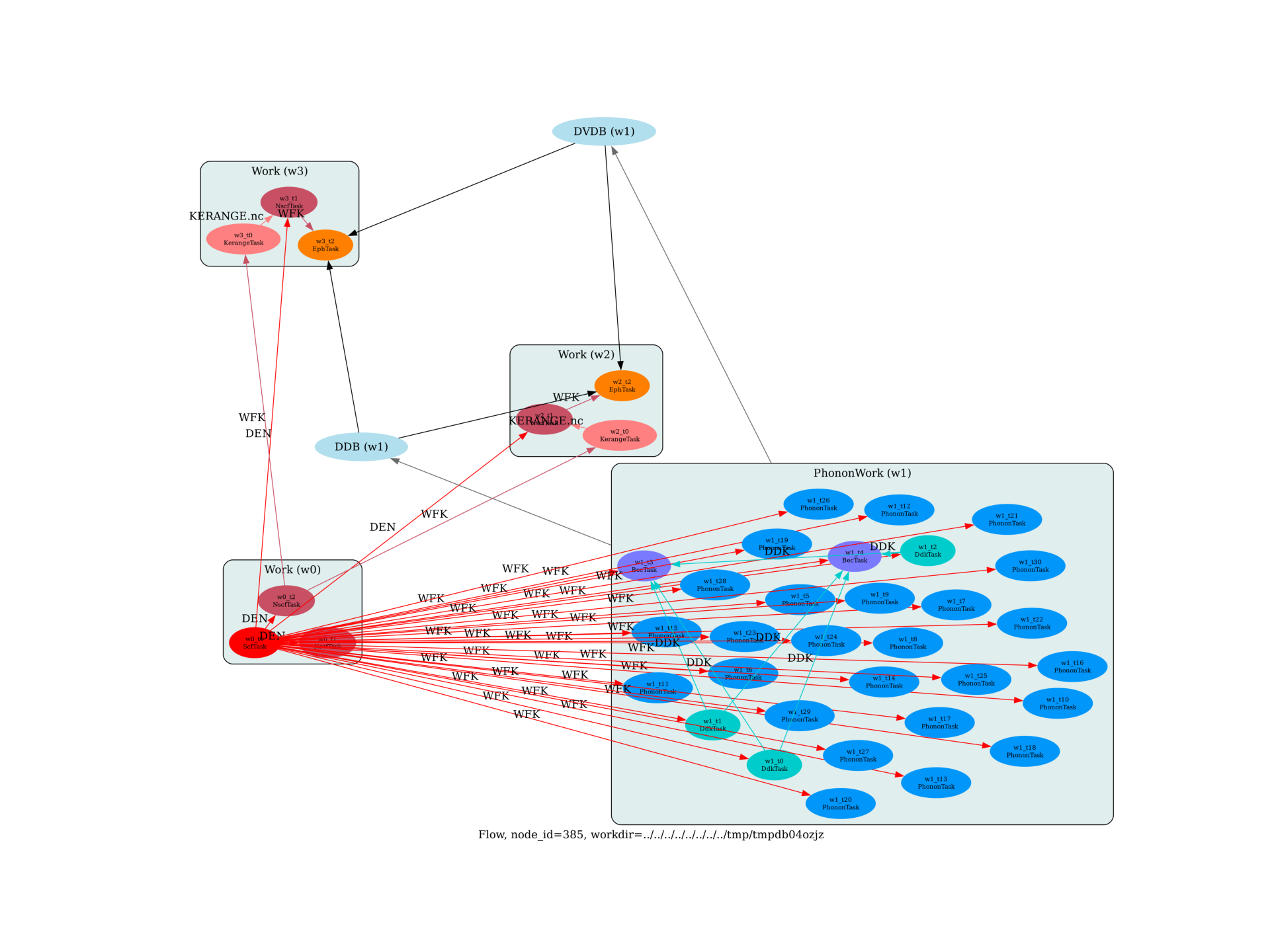Flow for phonon-limited mobilities in semiconductors¶

This flow computes the phonon-limited mobility in AlAs. using different dense k/q meshesOut:

<KerangeTask, node_id=438077, workdir=../../../../../../../var/folders/nc/k69spyd12qv2tk3stk2xrxg40000gr/T/tmpxl95dkcg/w2/t0>: setting prtwf to -1
<KerangeTask, node_id=438081, workdir=../../../../../../../var/folders/nc/k69spyd12qv2tk3stk2xrxg40000gr/T/tmpxl95dkcg/w3/t0>: setting prtwf to -1

import sys
import os
import abipy.data as abidata
import abipy.abilab as abilab
import abipy.flowtk as flowtk
import abipy.core.abinit_units as abu

def build_flow(options):

# Working directory (default is the name of the script with '.py' removed and "run_" replaced by "flow_")
if not options.workdir:
options.workdir = os.path.basename(sys.argv).replace(".py", "").replace("run_", "flow_")

# Initialize the flow
flow = flowtk.Flow(workdir=options.workdir, manager=options.manager)

# Initialize the tmesh, sigma_kerange, sigma_erange and dense meshes for the eph integrations
tmesh = [300, 300, 1]
sigma_kerange = [0, 0.5 * abu.eV_Ha] # 0.5 eV range for the WFK of electrons
sigma_erange = [0, 0.25 * abu.eV_Ha] # 0.25 eV range for the EPH computation for electrons
dense_meshes = [[30, 30, 30],
[40, 40, 40]]

# Initialize the structure and pseudos
structure = abidata.structure_from_ucell("AlAs")
pseudos = abidata.pseudos("13al.981214.fhi", "33as.pspnc")

# Ground-state computation for 1) the phonons and 2) the WFK generation
scf_input = abilab.AbinitInput(structure, pseudos=pseudos)

scf_input.set_vars(
nband=8,
ecut=2.0,
ngkpt=[4, 4, 4],
shiftk=[0, 0, 0],
tolvrs=1.0e-10,
diemac=9.0,
prtden=1,
#iomode=3,
)

work0 = flowtk.Work()

# Band structure calculation to make sure everything is OK
# Also allows to compare the results obtained with abitk to
# check the SKW interpolation works as needed
bs_input = scf_input.make_ebands_input(tolwfr=1e-12, ndivsm=10, nb_extra=5)
bs_input.set_vars(nstep=100, nbdbuf=1)

# NSCF input for the WFK needed to interpolate with kerange
nscf_input = abilab.AbinitInput(structure, pseudos)
nscf_input.set_vars(
ecut=2,
nband=8,
iscf=-2,
tolwfr=1e-20,
prtwf=1,
ngkpt=[16, 16, 16], # Should be dense enough so that the kerange interpolation works
shiftk=[0.0, 0.0, 0.0],
)

flow.register_work(work0)

# Add the phonon work to the flow
ddb_ngqpt = [4, 4, 4]
flow.register_work(ph_work)

# We loop over the dense meshes
for i, sigma_ngkpt in enumerate(dense_meshes):
# Use the kerange trick to generate a WFK file
multi = nscf_input.make_wfk_kerange_inputs(sigma_kerange=sigma_kerange,
sigma_ngkpt=sigma_ngkpt)
kerange_input, wfk_input = multi.split_datasets()

work_eph = flowtk.Work()
deps={work0: "DEN", work_eph: "KERANGE.nc"})

# Generate the input file for the transport calculation.
# Use ibte_prep = 1 to activate the iterative BTE.
eph_input = wfk_input.make_eph_transport_input(ddb_ngqpt=ddb_ngqpt,
sigma_erange=sigma_erange,
tmesh=tmesh,
eph_ngqpt_fine=sigma_ngkpt,
ibte_prep=1)

# We compute the phonon dispersion in the EPH code to be able to check they are ok.
if i == 0:
eph_input.set_qpath(20)

deps={work_eph: "WFK", ph_work: ["DDB", "DVDB"]})

flow.register_work(work_eph)

flow.allocate(use_smartio=True)

return flow

# This block generates the thumbnails in the AbiPy gallery.
# You can safely REMOVE this part if you are using this script for production runs.
__name__ = None
import tempfile
options = flowtk.build_flow_main_parser().parse_args(["-w", tempfile.mkdtemp()])
build_flow(options).graphviz_imshow()

@flowtk.flow_main
def main(options):
"""
This is our main function that will be invoked by the script.
flow_main is a decorator implementing the command line interface.
Command line args are stored in `options`.
"""

return build_flow(options)

if __name__ == "__main__":
sys.exit(main())

Total running time of the script: ( 0 minutes 2.711 seconds)

Gallery generated by Sphinx-Gallery